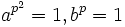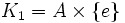# Permutability is not upper join-closed

This article gives the statement, and possibly proof, of a subgroup property (i.e., permutable subgroup) not satisfying a subgroup metaproperty (i.e., upper join-closed subgroup property).
View all subgroup metaproperty dissatisfactions | View all subgroup metaproperty satisfactions|Get help on looking up metaproperty (dis)satisfactions for subgroup properties
Get more facts about permutable subgroup|Get more facts about upper join-closed subgroup property|

## Statement

It is possible to have a group$G$, a subgroup$H$, and intermediate subgroups$K_1, K_2$ of$G$ containing$H$ such that$H$ is a permutable subgroup in both$K_1$ and$K_2$, but$H$ is not a permutable subgroup in$\langle K_1, K_2 \rangle$.

## Proof

### Construction of the counterexample

Setup: Let$p$ be an odd prime.

•$A$ is a group generated by two elements$a,b$ subject to the relations$a^{p^2} = 1, b^p = 1$ and$ab = ba^{p+1}$. Alternatively$A$ is the semidirect product of the additive group modulo$p^2$ by the multiplicative group of order$p$ in the multiplicative group of automorphisms. Note that$A$ is a non-Abelian group of order$p^3$.
•$C$ is a cyclic group of order$p^2$, generated by an element$c$.
•$G = A \times C$.
•$B = \{ b \}$.
•$K_1 = A \times \{ e \}$, and$K_2 = B \times C = \{b , c\}$.
•$H = B \times \{ e \}$.

We claim that$H$ is permutable in both$K_1$ and$K_2$ but not in$G$.

•$H$ is permutable in$K_1$: This follows from the fact that$B$ is permutable in$A$. This can be verified using the fact that it is in the Baer norm, or equivalently, that it commutes with all cyclic subgroups. The proof details are given in the example for permutable not implies normal.
•$H$ is permutable in$K_2$: This follows from the fact that$H$ is a direct factor of$K_2$, hence normal in$K_2$, hence permutable in$K_2$.
•$H$ is not permutable in$G$: Consider the cyclic subgroup$D$ generated by$(a,c)$. The claim is that$HD \ne DH$. To prove this notice that$DH \ni (a,c)(b,e) = (ab,c) = (ba^{p+1},c)$. This is clearly not in$HD$.